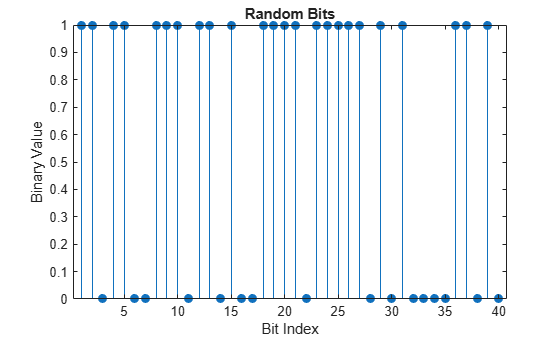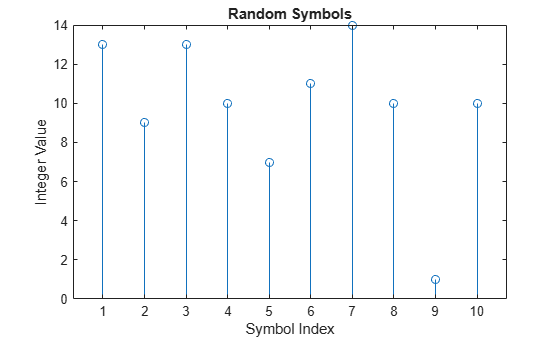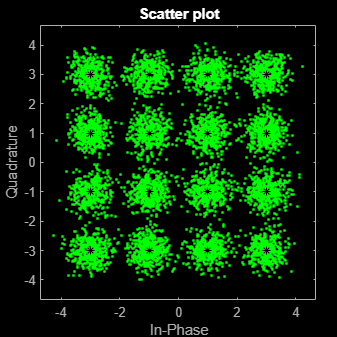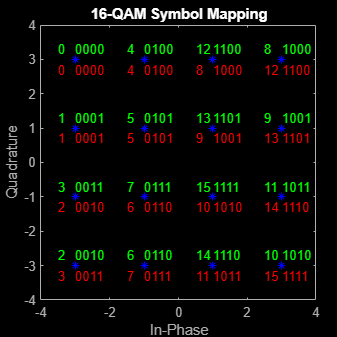Examine 16-QAM Using MATLAB

This example shows how to process a binary data stream by using a communications link that consists of a baseband modulator, channel, and demodulator. The example displays a portion of the random data in a stem plot, displays the transmitted and received signals in constellation diagrams, and computes the bit error rate (BER). To add a pulse shaping filter to the communications link, see the Use Pulse Shaping on 16-QAM Signal example. To add forward error correction to the communications link with pulse shape filtering, see the Use Forward Error Correction on 16-QAM Signal example.

Modulate Random Signal

The modulation scheme uses baseband 16-QAM, and the signal passes through an additive white Gaussian noise (AWGN) channel. The basic simulation operations use these Communications Toolbox™ and MATLAB® functions.

• rng — Controls the random number generation.

• randi — Generates a random binary data stream.

• bi2de — Converts the binary signal to an integer-valued signal.

• qammod — Modulates using 16-QAM.

• comm.AWGNChannel — Impairs the transmitted data using AWGN.

• scatterplot — Creates constellation diagrams.

• qamdemod — Demodulates using 16-QAM.

• de2bi — Converts the integer-valued signal to a binary signal.

• biterr — Computes the system BER.

Generate Random Binary Data Stream

The conventional format for representing a signal in MATLAB is a vector or matrix. The randi function creates a column vector containing the values of a binary data stream. The length of the binary data stream (that is, the number of rows in the column vector) is arbitrarily set to 30,000.

Define parameters.

M = 16; % Modulation order (alphabet size or number of points in signal constellation)
k = log2(M); % Number of bits per symbol
n = 30000; % Number of bits to process
sps = 1; % Number of samples per symbol (oversampling factor)

Set the rng function to its default state, or any static seed value, so that the example produces repeatable results. Then use the randi function to generate random binary data.

rng default;
dataIn = randi([0 1],n,1); % Generate vector of binary data

Use a stem plot to show the binary values for the first 40 bits of the random binary data stream. Use the colon (:) operator in the call to the stem function to select a portion of the binary vector.

stem(dataIn(1:40),'filled');
title('Random Bits');
xlabel('Bit Index');
ylabel('Binary Value');Convert Binary Signal to Integer-Valued Signal

The default configuration for the qammod function expects integer-valued data as the input symbols to modulate. In this example, the binary data stream is preprocessed into integer values before using the qammod function. In particular, the bi2de function converts each 4-tuple to a corresponding integer in the range [0, (M–1)]. The modulation order, M, is 16 in this example.

Perform a bit-to-symbol mapping by first reshaping the data into binary k-tuples, where k is the number of bits per symbol defined by $k=lo{g}_{2}\left(M\right)$. Then, use the bi2de function to convert each 4-tuple to an integer value.

dataInMatrix = reshape(dataIn,length(dataIn)/k,k);
dataSymbolsIn = bi2de(dataInMatrix);

Plot the first 10 symbols in a stem plot.

figure; % Create new figure window.
stem(dataSymbolsIn(1:10));
title('Random Symbols');
xlabel('Symbol Index');
ylabel('Integer Value');Modulate Using 16-QAM

Use the qammod function to apply 16-QAM modulation to the dataSymbolsIn column vector for natural-encoded and Gray-encoded binary bit-to-symbol mappings.

dataMod = qammod(dataSymbolsIn,M,'bin'); % Binary coding with phase offset of zero
dataModG = qammod(dataSymbolsIn,M); % Gray coding with phase offset of zero

The modulation operation outputs complex column vectors containing values that are elements of the 16-QAM signal constellation. Later in this example constellation diagrams show the natural and Gray binary symbol mapping.

For more information on modulation functions, see Digital Modulation. For an example that uses Gray coding with phase-shift keying (PSK) modulation, see Gray-Coded Binary Ordering.

The modulated signal passes through the channel by using the awgn function with the specified signal-to-noise ratio (SNR). Convert the ratio of energy per bit to noise power spectral density (${\mathit{E}}_{\mathrm{b}}/{\mathit{N}}_{0}$) to an SNR value for use by the awgn function. The sps variable is not significant in this example but makes extending the example to use pulse shaping easier. For more information, see the Use Pulse Shaping on 16-QAM Signal example.

Calculate the SNR when the channel has an ${\mathit{E}}_{\mathrm{b}}/{\mathit{N}}_{0}$ of 10 dB.

EbNo = 10;
snr = EbNo+10*log10(k)-10*log10(sps);

Pass the signal through the AWGN channel for the binary and Gray coded symbol mappings.

Create Constellation Diagram

Use the scatterplot function to display the in-phase and quadrature components of the modulated signal, dataMod, and the noisy signal received after the channel. The effects of AWGN are present in the constellation diagram.

hold on
scatterplot(dataMod,1,0,'k*',sPlotFig)Demodulate 16-QAM

Use the qamdemod function to demodulate the received data and output integer-valued data symbols.

Convert Integer-Valued Signal to Binary Signal

Use the de2bi function to convert the data symbols from the QAM demodulator, dataSymbolsOut, into a binary matrix, dataOutMatrix, with dimensions ${\mathit{N}}_{\mathrm{sym}}$–by–${\mathit{N}}_{\mathrm{bits}/\mathrm{sym}}$. In the matrix, ${\mathit{N}}_{\mathrm{sym}}$ is the total number of QAM symbols, and ${\mathit{N}}_{\mathrm{bits}/\mathrm{sym}}$ is the number of bits per symbol. For 16-QAM, ${\mathit{N}}_{\mathrm{bits}/\mathrm{sym}}$ = 4. Then convert the matrix into a column vector of length equal to the number of input bits, 30,000. Repeat the process for the Gray-encoded data symbols, dataSymbolsOutG.

Reverse the bit-to-symbol mapping performed earlier in this example.

dataOutMatrix = de2bi(dataSymbolsOut,k);
dataOut = dataOutMatrix(:); % Return data in column vector
dataOutMatrixG = de2bi(dataSymbolsOutG,k);
dataOutG = dataOutMatrixG(:); % Return data in column vector

Compute System BER

The biterr function calculates the bit error statistics from the original binary data stream, dataIn, and the received data streams, dataOut and dataOutG. Gray coding significantly reduces the BER.

Use the error rate function to compute the error statistics. Use the fprintf function to display the results.

[numErrors,ber] = biterr(dataIn,dataOut);
fprintf('\nThe binary coding bit error rate is %5.2e, based on %d errors.\n', ...
ber,numErrors)
The binary coding bit error rate is 2.40e-03, based on 72 errors.
[numErrorsG,berG] = biterr(dataIn,dataOutG);
fprintf('\nThe Gray coding bit error rate is %5.2e, based on %d errors.\n', ...
berG,numErrorsG)
The Gray coding bit error rate is 1.33e-03, based on 40 errors.

Plot Signal Constellations

The constellation diagram shown previously plotted the points in the QAM constellation, but it did not indicate the mapping between symbol values and the constellation points. In this section, the constellation diagram indicates the mappings for natural-encoding and Gray-encoding of binary data to constellation points.

Show Natural and Gray Coded Binary Symbol Mapping for 16-QAM Constellation

Apply 16-QAM modulation to complete sets of constellation points by using natural-coded binary symbol mapping and Gray-coded symbol mapping.

M = 16; % Modulation order
x = (0:15); % Integer input
symbin = qammod(x,M,'bin'); % 16-QAM output (natural-coded binary)
symgray = qammod(x,M,'gray'); % 16-QAM output (Gray-coded)

Use the scatterplot function to plot the constellation diagram and annotate it with natural (red) and Gray (black) binary representations of the constellation points.

scatterplot(symgray,1,0,'b*');
for k = 1:M
text(real(symgray(k)) - 0.0,imag(symgray(k)) + 0.3,...
dec2base(x(k),2,4));
text(real(symgray(k)) - 0.5,imag(symgray(k)) + 0.3,...
num2str(x(k)));

text(real(symbin(k)) - 0.0,imag(symbin(k)) - 0.3,...
dec2base(x(k),2,4),'Color',[1 0 0]);
text(real(symbin(k)) - 0.5,imag(symbin(k)) - 0.3,...
num2str(x(k)),'Color',[1 0 0]);
end
title('16-QAM Symbol Mapping')
axis([-4 4 -4 4])Examine Plots

Using Gray-coded symbol mapping improves BER performance because the Gray-coded signal constellation points differ by only one bit from each adjacent neighboring point. Where as with natural binary symbol mapping, some of the adjacent constellation points. For example, the natural-coded binary values for 1 (0 0 0 1) and 2 (0 0 1 0) differ by two bits (the third and forth bits).